Learn US

# The Beauty of Algebra

377 views

 1 Introduction 2 History of Algebra 3 Algebra Basics 4 How to Do Algebra 5 What Is Algebra Good For In Real Life? 6 The Application of Algebra 7 Conclusion 8 Frequently Asked Questions (FAQs) 9 External References

December 16, 2020

## Introduction

"The pure mathematician, like the musician, is a free creator of his world of ordered beauty." -Bertrand Russel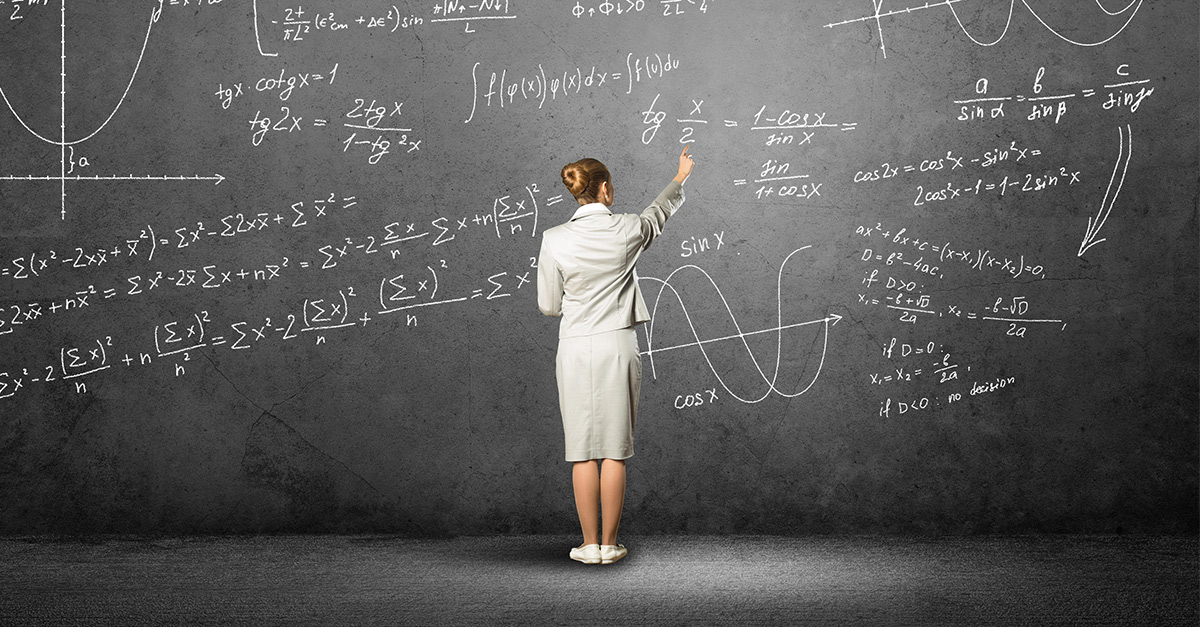The beauty of algebra lies in its equations; each of the equations defines the relationship between X, Y, and Z. Relationships and connections are all around us.

• Algebra is not about memorizing formulas or manipulating complicated expressions or long lists of functional properties. It's a way of organizing one's thinking—taking complex problems and breaking them into pieces, exploring possible solutions, and formulating solutions, so they apply to other complex problems in the future.

• Algebra isn't facts and procedures—it's thinking, and understanding and those skills are valuable, carrying over to real-life; plenty of evidence demonstrates this (for example, How people learn, published by the National Academies of Science).

• Algebra reinforces logical thinking and is the underlying mechanism of most advanced algorithms such as  Machine Learning, Deep Learning, Artificial intelligence. Also, Linear Algebra helps in boosting many programming languages.

• Algebra acts as a substrate to describe so many types of real-world examples from gravity to population growth. Algebra can be used to form big Matrices- (is a relationship which is all around us )and defines how an entire category of matter, namely ideal gases, behaves, also plays a significant role in Statistics, is impressive and beautiful in its simplicity.

### A science of solving equations

It is a branch of mathematics where we use the alphabet as a substitute for numbers.

In an algebraic equation, LHS should always be equal to RHS; it means that when a mathematical operation is done on one side of the equation, the same operation should be done on the other side of the equation, and the numbers act as constants. Algebra includes real numbers, complex numbers, matrices, vectors, and many other forms of mathematical representation.

## History of Algebra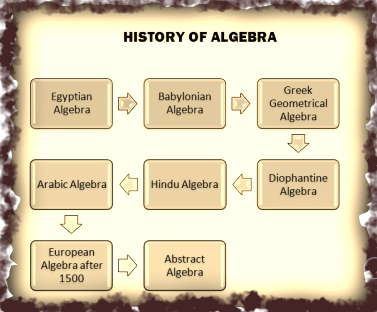Algebra was first introduced to the world by the Greeks and the Babylonians over 18 centuries ago. In the third century, they devised a system that helped to solve problems with both linear and quadratic equations. In layman terms, it helped them to simplify guess work and to make predictions for everyday tasks.

Guesswork has always existed intuitively as people constantly used known variables to make predictions about an unknown variable which it had a relationship with. The difference with algebra was that it replaced numbers with symbols. Instead of having to wrap your head around large ambiguous numbers, simple symbols were used in their place.

### A Simple Example of Algebra’s Value

Instead of stating: “I am finding a number which when multiplied by 8 before having an addition of 2, would give me 50”.

You could now simply rephrase it as “8x + 2 = 50, where x is an unknown number”.

When working with larger sums, complex numbers, and convoluted equations, these symbols allow us to simplify the problems for our minds.

## Algebra Basics

The Basics of Algebra include simple mathematical operations like addition, subtraction, multiplication, and division involving both constant as well as variables.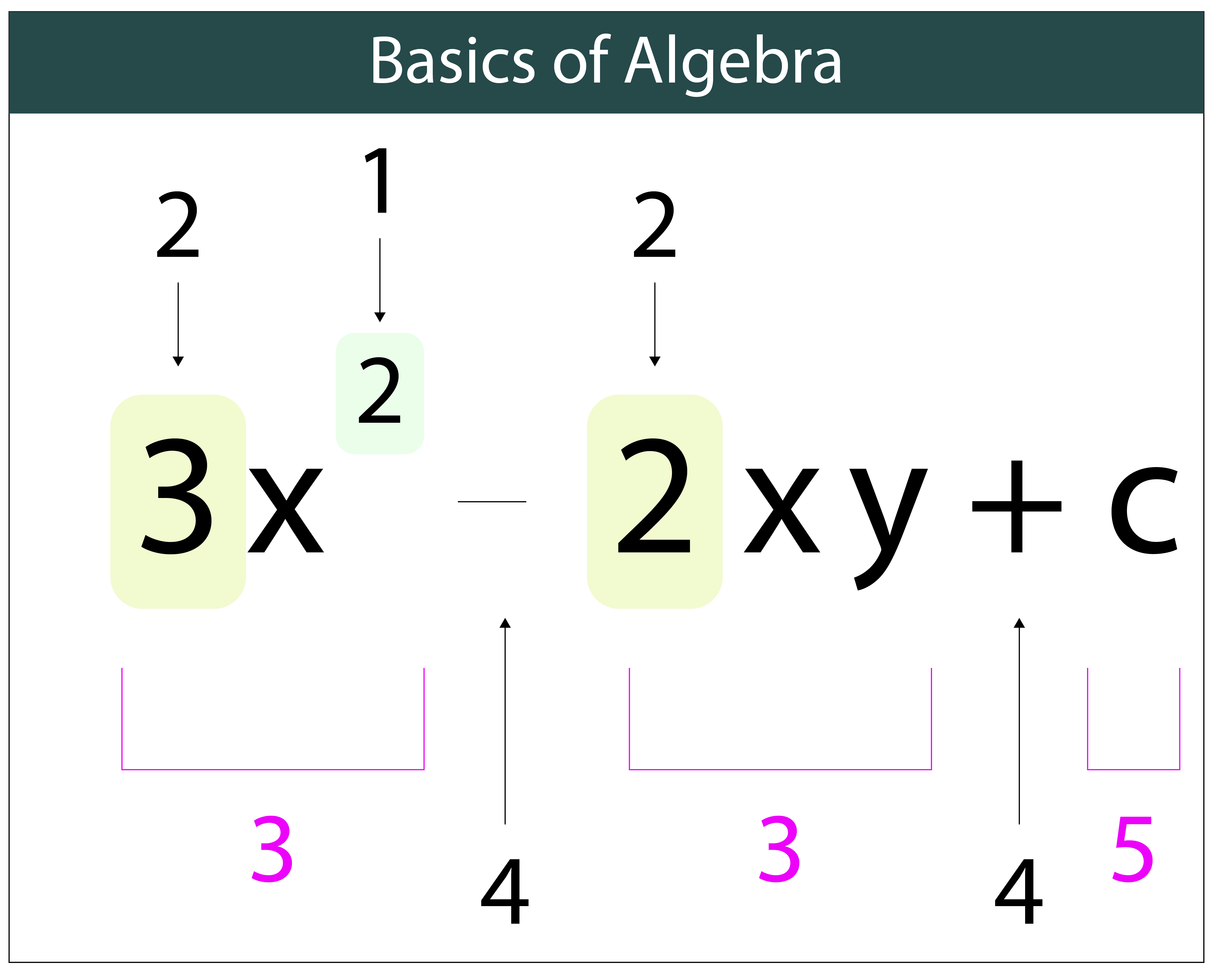In the given equation, the x and y represent unknown variables which have to be determined.
Whereas 3 and 2 are the numerical values.
C  --is the constant term.

### Algebraic Expression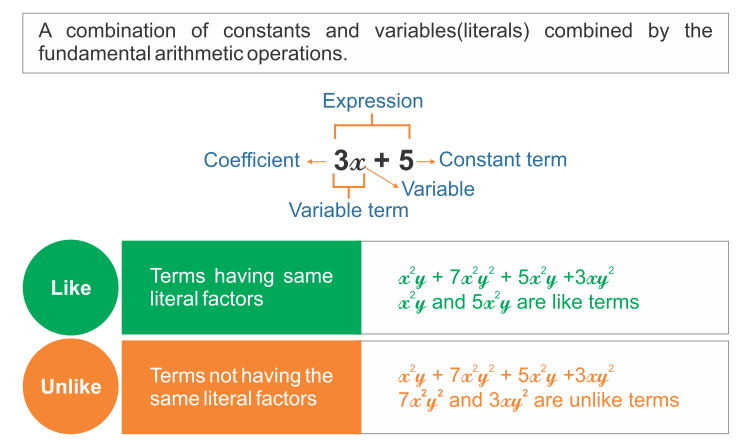Terms related to basic algebra are as follows:

1. Exponent
2. Expression
3. Polynomial (Monomial, binomial and trinomial)
4. Like terms and Unlike terms
5. Constants

An equation is a statement that consists of two same identities separated by the “=” sign. Whereas an expression is a group of different terms separated by ‘+’ or ‘-‘ sign.
Like terms are those terms whose variables and their exponents are the same.

###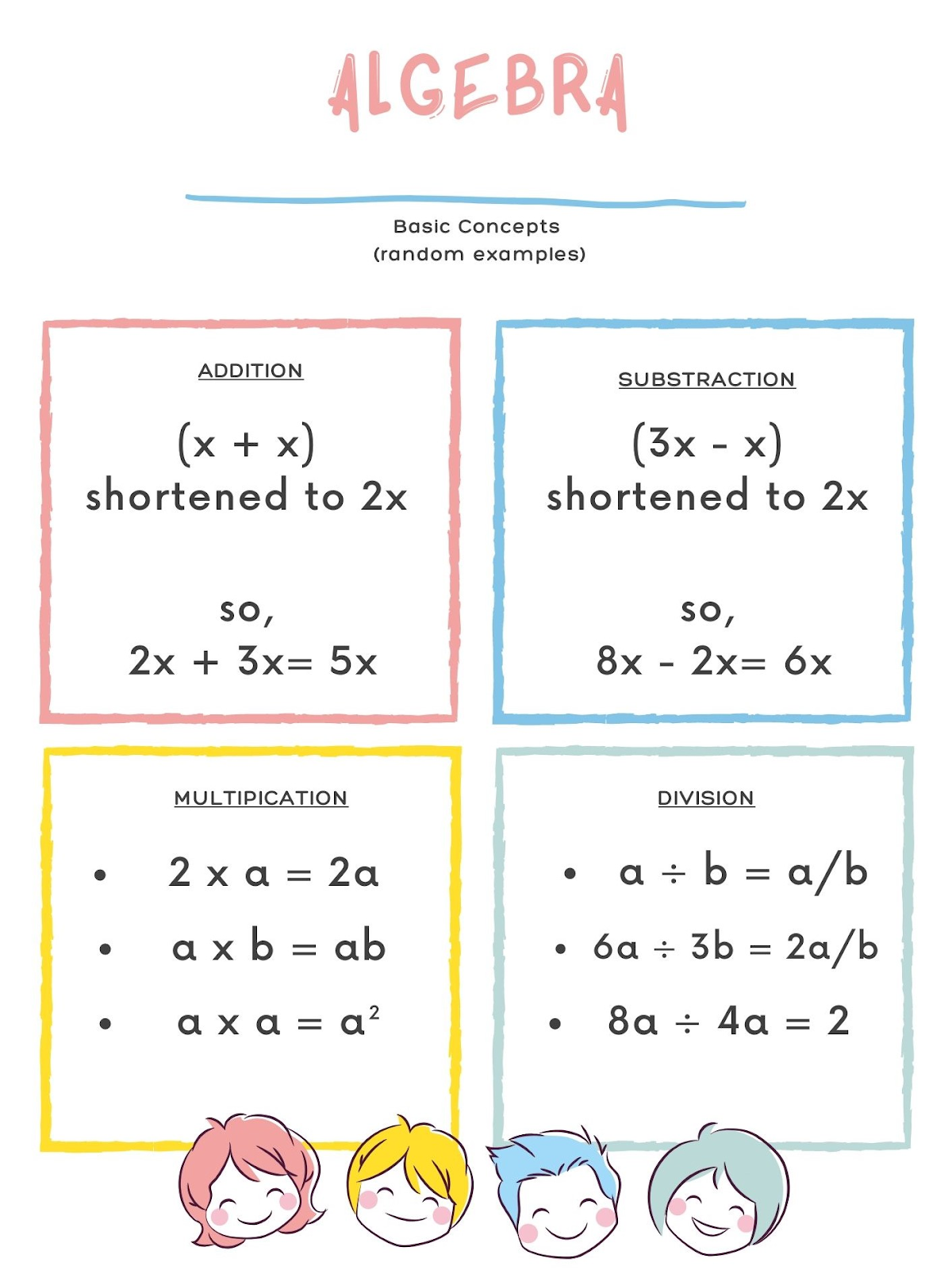The general arithmetic operations performed in algebra are:

1. Addition: ( x + y )
2. Subtraction:  ( x  –  y )
3. Multiplication: ( x × y  )
4. Division: ( x/y or x ÷ y)  Where x and y are the variables.

The order of these operations will follow the BODMAS rule, which means the terms inside the brackets are considered first. Then, roots and exponents are operated on second priority, followed by Solving all the division and multiplication operations and later addition and subtraction.

### Algebraic  Formulas

The general formulas used in algebra to solve algebraic equations and find the values of unknown variables are given here: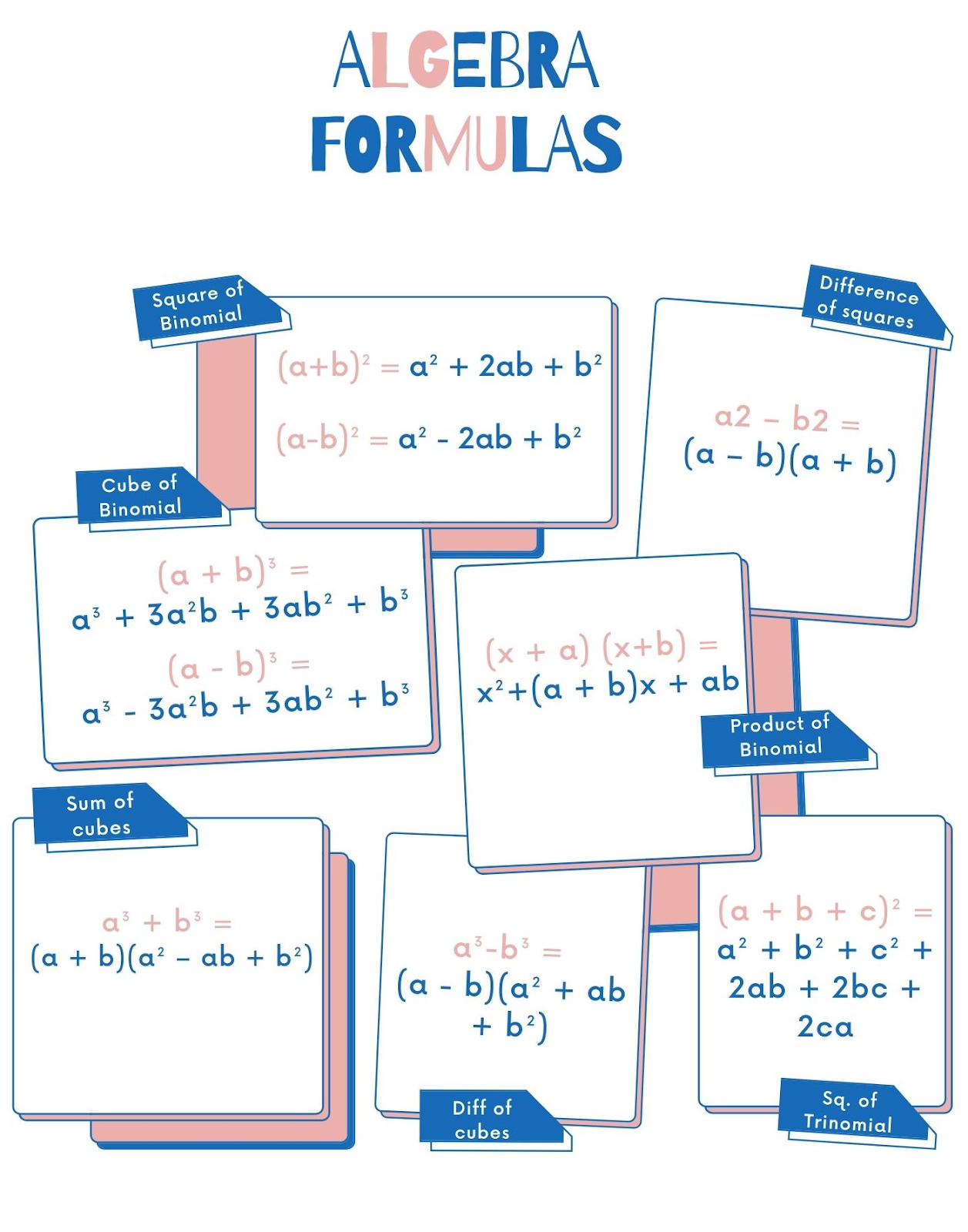### Algebraic  Rules

The basic algebraic rules are as follows:

• The Symmetry rule
• The commutative rules
• Two rules for equation

## How to Do Algebra

Algebra can be a difficult subject to master. In addition to numbers, there are letters thrown into equations. These letters are called variables, and they represent unknown numbers. It may seem overwhelming at first, but by learning a few basic concepts and doing practice problems, you can be successful in algebra. Once you have learned the basics, you will begin to see how useful algebra is, and applies to situations in everyday life!

### A. Understanding Order of Operations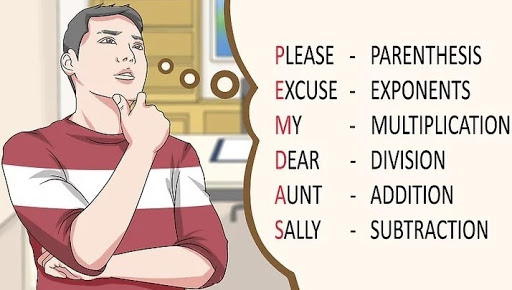1. Memorize PEMDAS

PEMDAS is an acronym to help you remember the order of operations in math. PEMDAS stands for Parentheses, Exponents, Multiplication and Division, Addition and Subtraction. Whenever you are solving a problem, start with the expressions in parentheses and work your way through the acronym, finishing with subtraction.

• For parentheses, perform all of the operations inside the parentheses using this same order.

• Multiplication and division are considered equal operations. You can solve them at the same time, so simply solve from left to right.

• Addition and subtraction are also equal operations, so solve from left to right.

• You can remember PEMDAS using the mnemonic, Please Excuse My Dear Aunt Sally.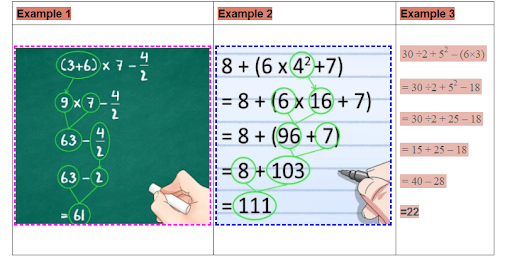For example, in the above equations

• The expression in parentheses was first solved
• Next, solved exponents
• Next, multiply and divide left-to-rightWhen starting to learn algebra, the material can get overwhelming very quickly. Don’t be afraid to ask your teacher for help or seek out extra tutoring. Even asking a friend who may have a better understanding can be useful.Ask your parents about getting a tutor if you are really struggling.

3.  Isolate the variable on one side of the equation

When given algebraic expression, you will notice that there are constants and variables. A constant is any number given, while a variable is a letter that represents an unknown number.
To isolate the variable, add or subtract terms to get the variable on one side. If the variable has a coefficient, divide both sides by that coefficient to get the variable alone

 Example

to solve 6y + 6 = 48, you first need to subtract 6 from both sides, then divide by 6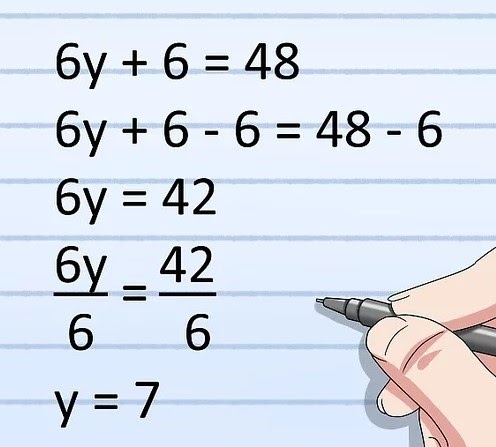4. Take the root of the number to cancel an exponent

If you are solving for a variable that is squared, you will need to take the square root of it to solve the problem. Conversely, If the variable is a square root, then you will need to square it to solve the problem. Remember that whatever you do to one side of the equation, you must do to the other side.

 Example:

1. to solve x = 9, you need to square both sides of the equation.

2. to solve x2= 16, you need to take the square root of both sides of the equation:

###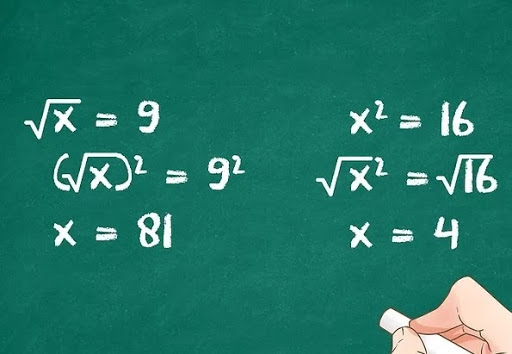5. Combine like terms

Whenever you have terms that have the same variable, you can combine them to simplify the problem. This helps to keep equations manageable and easier to solve. Remember, terms that have different exponents are not identical terms

x is not the same as x2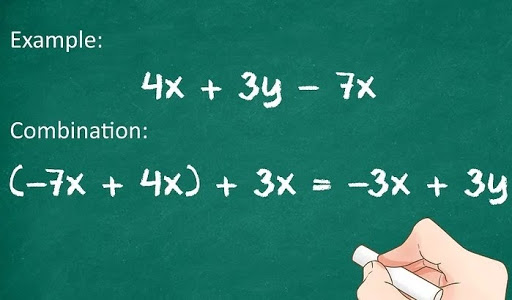• The following are like terms:

4x, -3x, 0.45x, -132x

• The following are not like terms:

5x,8y2,-13y,9z,12xy

 Example:

4x+3y-7x  has two like terms, 4x and  -7x.

(-7x + 4x) + 3y

= (-3x) + 3y

6. Practice with more complex problems.

The art of mastering any concept is practice. Try solving problems with increasing difficulty to truly check your comprehension. Use problems from your textbook or seek out extra problems online.

 Example

to solve q+18 = 9q - 6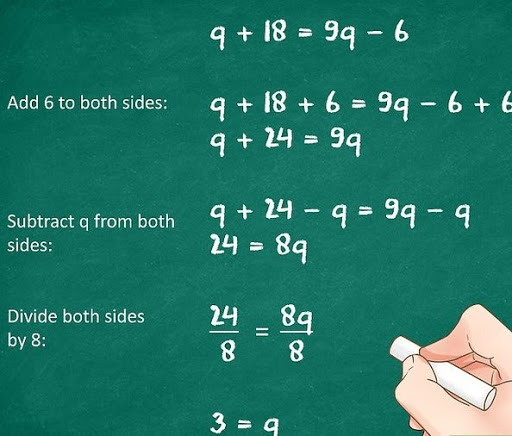Make a habit of checking your answers when you have solved a problem. Once you have obtained the solution and discovered the value of the variable, check your work by inserting the number you have obtained into the

original equation. If the expression is still true, then you have found the correct solution!

 Example:

substitute 3 for q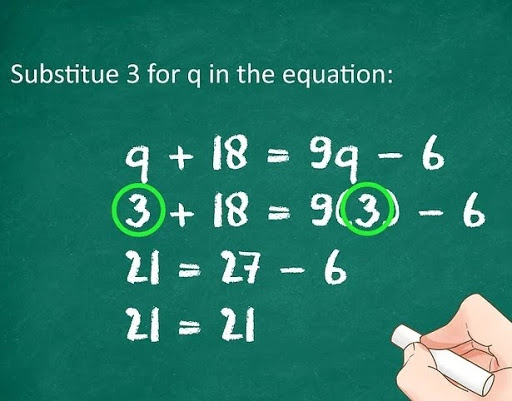Right! Since the equation is true, you know that your solution is correct.

### B. Solving Problems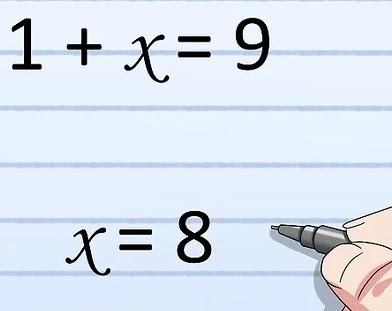• Recognize that algebra is just like solving a puzzle.

Like any puzzle, there are pieces. Learning how to recognize the numbers and symbols for the placeholders that they are makes the solution much easier to grasp.

• Try to find the missing number in a problem where the final answer is given. For example: 1+x=9
• The missing number is 8, because 1 plus 8 equals 9. Pretty simple, right? This is basic algebra.

• Perform operations on both sides of the equation.

When solving an algebraic problem, you must remember that if you alter one side of the equation in any way, you must do the exact same thing to the other side of the equation. If you add, subtract, multiply, or divide, you must perform the same operation to the opposite side.

 Example:

To solve  x + 3 = 2x - 1, Subtract x from both sides of the equation, then add 1 to both sides of the equation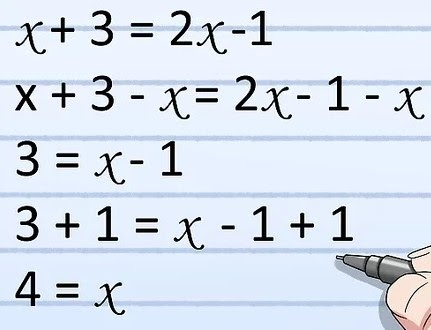### C. Multiplying with the FOIL Method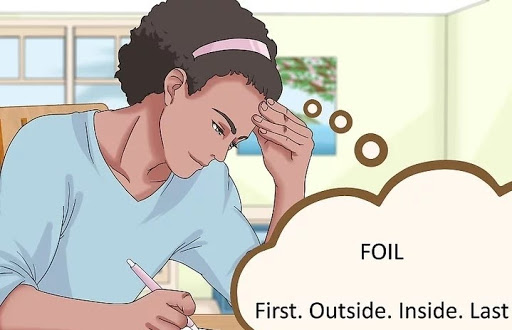1. Define FOIL. The FOIL method stands for First, Outside, Inside, Last. It is a method used to multiply two binomials together. A binomial is an algebraic expression with two terms, like 5x-3

For example, to calculate (5x-3)(4x+1) we have to use the FO.

2. Multiply the first terms of each binomial.

The “F” in FOIL stands for “First.” The first terms are the terms on the left in each set of parentheses. Remember that when you multiply two of the same variables together, the result is the variable, squared.

 Example: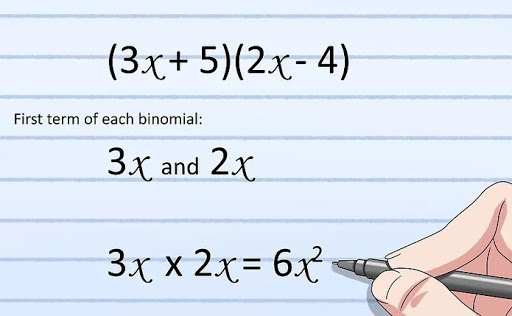3.Multiply the two outside terms together.

The “O” in FOIL stands for “Outside.” The outside term of the first binomial is on the left; the outside term of the second binomial is on the right.

 Example: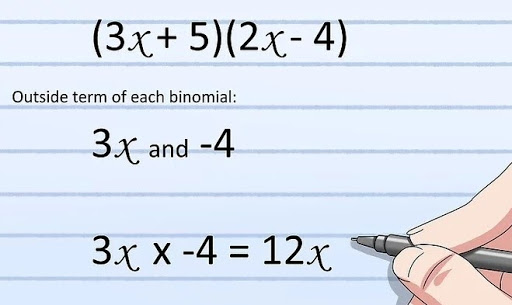4. Find the product of the two inside terms.

The “I” in FOIL stands for “Inside.” The inside term of the first binomial is on the right; the inside term of the second binomial is on the left.

 Example: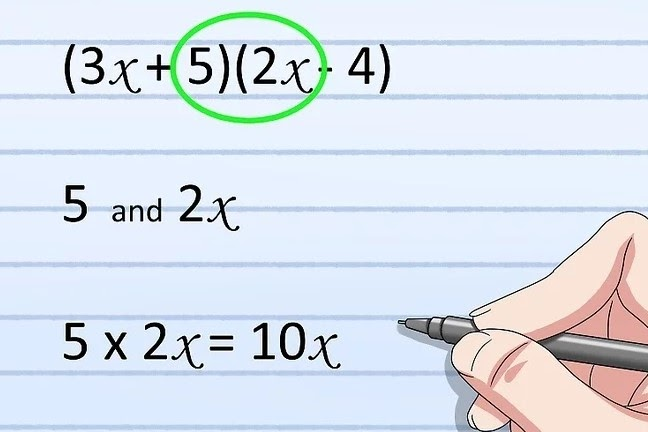5. Multiply the last two terms together.

The “L” in FOIL stands for “Last.” The last term of each binomial is on the right.

 Example: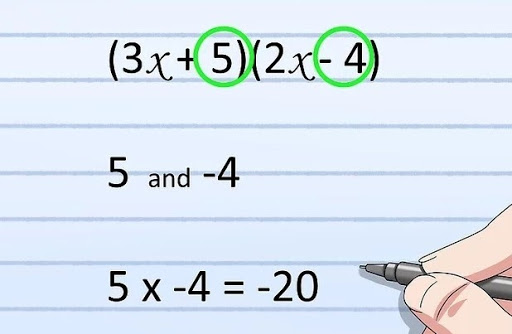6.Combine all terms and simplify.

After putting the expression together, you can combine like terms to simplify the expression fully. Make sure you pay close attention to positive and negative signs when adding like terms.

 Example:

Solve   (3x+5)(2x-4)

To solve  (3x+5)(2x-4) this equation is calculated as follows, after combining like terms simplify it.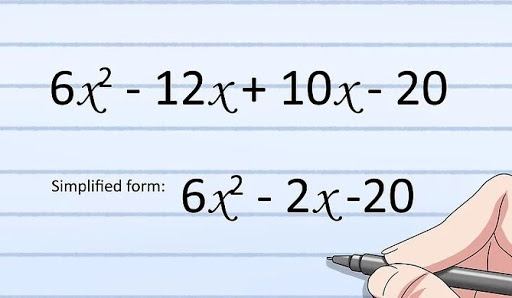D. Working with Exponents

1.Simplify exponents of numbers.

When a number has an exponent that means you multiply that number by itself as many times as the exponent says. To simplify any number that has an exponent simply multiply it the appropriate number of times.

 Example :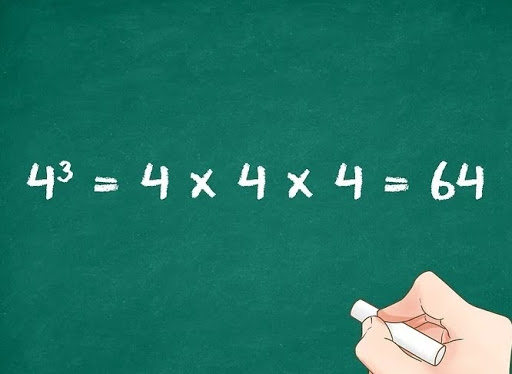•  If there is a negative sign and no parentheses, the exponent is simplified and then the negative sign gets added:
 Example:

-22=-(2*2)=-4

• If there is a negative sign, but the number is in parenthesis, the negative number is part of the exponent.
 Example:

(-2)2=-2*-2=-4

2.Combine like terms with the same exponents.

It may be confusing at first to see a variable with an exponent. Just remember, any variable with the same exponent number can be added or subtracted. If the letters are the same, but the exponents are different, they cannot be combined.

 Example: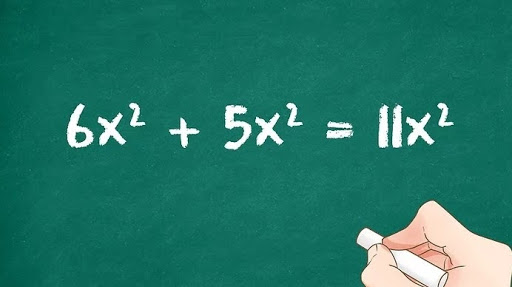In the above example, the equation is simplified as both the variables have an exponent.

On the other hand, 5z+5z2 cannot be simplified, since one variable has an exponent, and one does not.

3.Add the exponents together when multiplying variables.

If two variables are being multiplied together and they both have exponents, you can add the exponents together to get the resulting exponent. This only applies to variables of the same letter.

 Example: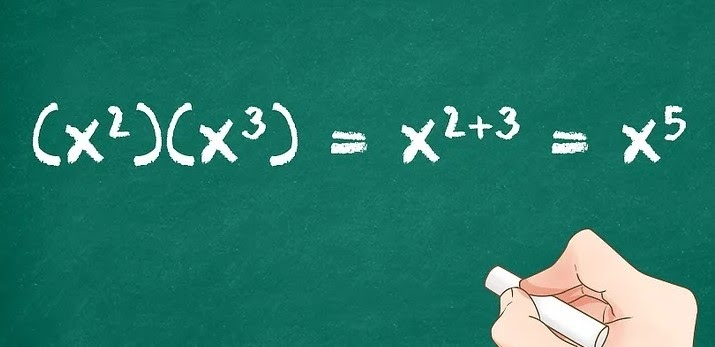4.Subtract the exponents when dividing variables.

If you want to divide two variables that have exponents, simply subtract the bottom exponent from the top exponent. This only applies to variables that are the same letter.

 Example: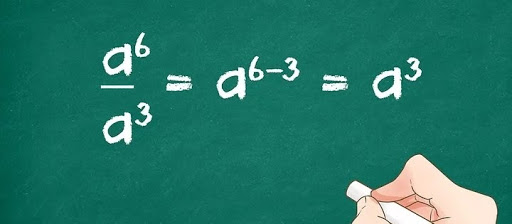## What Is Algebra Good For In Real Life?

Algebra has long been feared by students taking mathematics. Other than the perception of complexity, students also have trouble as they view it as an abstract topic. Without obvious real-life applications, students struggle to make sense of the symbols and equations used.

Yet, algebra at its core was invented as a tool to help us solve problems that existed in the real world. As such, the value of algebra can only be understood by first looking at its origins.

## The Application of Algebra

For most students, mention the application of algebra and we think of concepts such as:

• Simultaneous equations
• Differentiation & Integration
• Probability
• Trigonometry
• Binomial theorem

All of these concepts do use algebra to express their various relationships and formulas. This makes algebra an integral part of them. However, these concepts again remain largely abstract in students’ eyes.
To give context of the usefulness of algebra beyond your school, here we collected a list of examples where it is utilised.

1.Algebra in Our Everyday Activities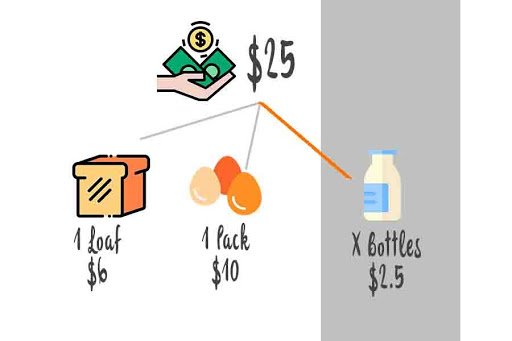Budgeting in a Supermarket

Algebra is intuitively used in daily budgeting. Take the example of a grocery trip to a supermarket.

If you had a budget of $25 and wanted to buy bread ($6/loaf), eggs ($10/pack) & milk ($2.5/bottle). Assuming the following conditions:

• You need 1 loaf of bread
• You need 1 pack of eggs
• You want as many bottles of milk as possible

A simple equation can be used to determine how much milk to buy:

25 = 6 + 10 + 2.5x
where x is the number of milk bottles.

While most people will not explicitly create this equation in their minds, they do intuitively express it in a different form when calculating:

Essentially, you would subtract the cost of bread ($6) and eggs ($10) from your max budget ($25), before dividing the remainder by the cost of a bottle of milk ($2.5) to find the number of milk bottles (x).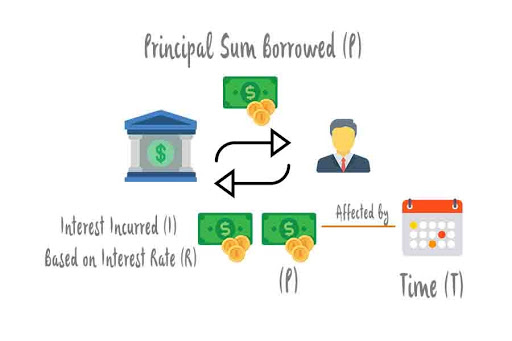A. Bank Interest on Loans

When you secure a loan from a bank, you will most likely be required to pay back more than the principal borrowed. This is due to the incurring of interest over the duration of the loan. The simple interest amount can be calculated based on the following formula: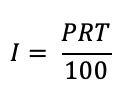I: Simple interest
P: Principal amount borrowed
I: Interest rate, which is set by the bank
T: Duration

Calculating and projecting the simple interest incurred on a loan will help you to determine the duration in which is optimal for you to repay the loan within.

All businesses incur cost in the production of goods which are required in order for a profit to be made from their sale. Through careful planning and forecast, a greater profit can be earned.

The cost and corresponding profit per unit are not constant due to the spreading out of fixed costs among the units produced. As such, it is useful to project the business revenue into the future based on the existing data by using Algebra.

Based on the daily revenue over a period of 1 month, the business owner derives the Revenue formula:
R = 11×2 – 500x + 30000

R: Revenue earned for day x

x: exact day of operation

We can forecast the revenue of the subsequent month by the carrying out the following algebra manipulation: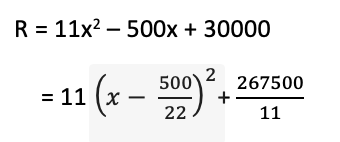From the equation,
on day  = 22.7272,
the forecasted revenue will be the lowest at  = \$24318.18182.

Hence, the business owner will be able to control cost on that specific day by cutting down on the manpower and operating expenses.  This in turn helps to generate the highest profit possible and make sound decision on the

3.Algebra in Computer Programming

Our final example of algebra involves an indirect but complex application in the world of computer programming. For clarity, you do not need algebra to perform programming.

However, an “algebraic” way of thinking will be of great assistance in coding.

Why do we say that? Programming involves the usage of abstract rules (an equation) to automate the creation of the end result. Within the stated rule, lies various variables which can be expressed as symbols not unlike what

we have covered thus far.

Computer programming employs the same type of logic as found in algebra, however since it is intended for machine automation, further rules are added on. These rules can be thought of an expansion of the base formula.

As such, students who are confident in algebra tend to pick up programming logic at a relatively quicker pace.### Einstein’s Takeaway

Algebra is applied intuitively or intentionally to solve a host of different problems in the real world. Even where it is not directly applicable, having trained your mind to be comfortable with algebra, you will find success with many other similar concepts.

## Conclusion

Remember that Algebra is the beginning of abstract thinking in mathematics. Hence it is imperative that we understand what model they have in their mind and then help to shape that model. Most important thing is to remove the fear of the use of alphabets in mathematical expressions.

Make it fun by using puzzles and then inculcate the understanding of function representation. Then, definitions around like terms, coefficients, and others will naturally follow.

Remember that Algebra is the beginning of abstract thinking in mathematics. Hence, it is imperative that we understand what is the model they have in their mind and then help shape that model.

Most important is to remove the fear of the use of the alphabet in mathematical expressions. Make it fun by using puzzles and then inculcate the understanding of function representation.

Then, definitions around the like terms, coefficients, and others will naturally follow.

Cuemath, a student-friendly mathematics and coding platform, conducts regular Online Live Classes for academics and skill-development, and their Mental Math App, on both iOS and Android, is a one-stop solution for kids to develop multiple skills. Understand the Cuemath Fee structure and sign up for a free trial.

## 1. Is Linear Algebra easy?

Conceptually Linear Algebra is very hard, but the mechanics aren't hard.